# BMI by Country

Estimated mean Body Mass Index (BMI) trend by country

Estimated mean Body Mass Index (BMI) trend between 1975 and 2014 by country.

BMI (kg/m2) estimates are from male/female populations over the age of 18.

## Examples

### Basic Examples

Retrieve the resource:

 In:=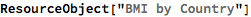Out=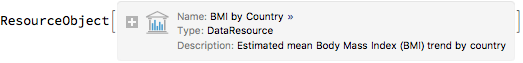Retrieve the default content:

 In:=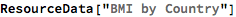Out=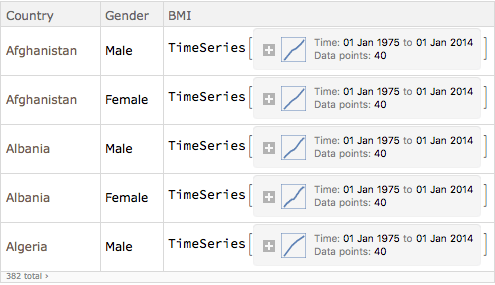Plot estimated BMI of G7 (Group of 7) countries over time:

 In:=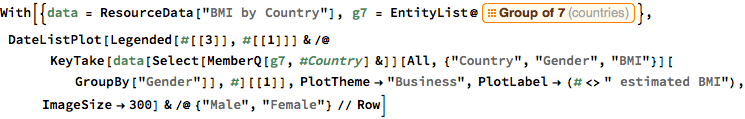Out=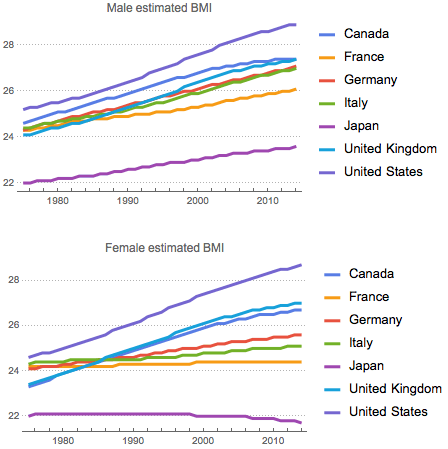Color countries by estimated BMI:

 In:=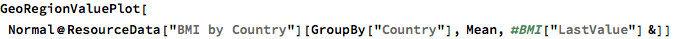Out=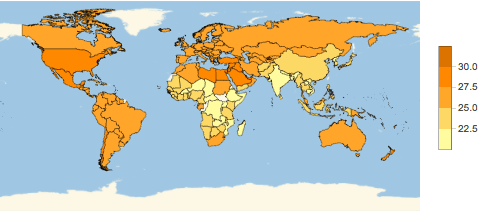Wolfram Research, "BMI by Country" from the Wolfram Data Repository (2017) https://doi.org/10.24097/wolfram.65128.data